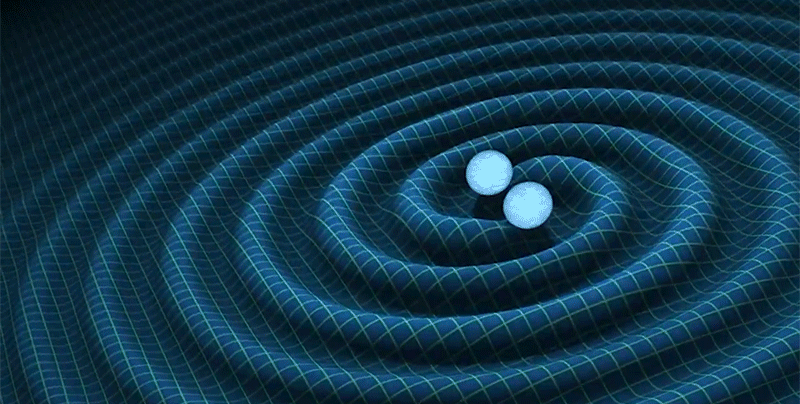# Mathematical Relativity — 2nd Semester 2021/2022

 Lecturer: José Natário Email: jnatar@math.tecnico.ulisboa.pt Office: Mathematics Building, 4th floor, room 4.29 Classes: Tuesdays and Thursdays from 9:00 to 11:00. Office Hours: Drop by my office or send me an email.

## Announcements

You can see the final grades after the second exam on the side bar. Drop by my office if you want to take a look at your graded exam.

## Syllabus

Examples: Minkowski space-time, Schwarzschild solution, Einstein, de Sitter, anti-de Sitter and Friedman-Lemaitre-Robertson-Walker universes; matching and Oppenheimer-Snyder collapse; Penrose diagrams.

Causality: time orientabiliy, chronological and causal past and future, domain of dependence; chronological, stably causal and globally hyperbolic spacetimes.

Singularities: Jacobi equation and conjugate points; energy conditions; existence of maximizing geodesics; Hawking and Penrose singularity theorems.

Cauchy Problem: wave equation; Cauchy problems with constraints; Gauss-Coddazzi relations and 3+1 decomposition of the Einstein equation; Choquet-Bruhat theorem; constraint equations for the initial data.

Positive Mass Theorem: Komar mass; Einstein-Hilbert action; Lagrangian and Hamiltonian formulation of the Einstein equations; ADM mass; positive mass theorem; Penrose inequality.

Black Holes: Kerr solution; Killing horizons; surface gravity; Smarr formula; area theorem; black hole thermodynamics.

## Bibliography

Main

Secundary

Homework: Makes up 50% of the grade. Late homework will not be accepted.

Final exam: Makes up 50% of the grade. Can be retaken if necessary.

## Homework

• Homework 1 (due on March 24): Exercise 1 in Chapter 2
• Homework 2 (due on April 5): Exercise 8 in Chapter 2
• Homework 3 (due on April 19): Exercise 7 in Chapter 3
• Homework 4 (due on May 17): Exercises 2 and 4 in Chapter 4
• Homework 5 (due on May 26): Exercises 7, 8 and 10 in Chapter 4
• Homework 6 (due on June 9): Exercises 1 and 4 in Chapter 5
• Homework 7 (due on June 21): Exercises 5 and 6 in Chapter 5
• Homework 8 (due on June 30): Exercises 5 and 8 in Chapter 6